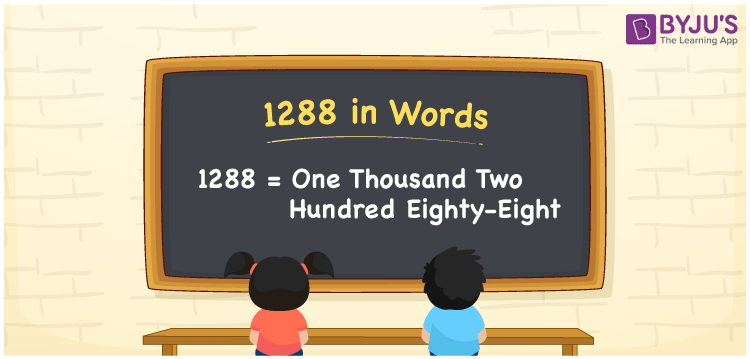# 1288 in Words

1288 in words is one thousand two hundred eighty-eight. The number 1288 appears after 1287 but before 1289. Also, 1288 is a cardinal number. For example, if you spent Rs. 1288 on purchasing toys, you can write as “I spent Rs. One thousand two hundred eighty-eight on purchasing toys”.

 1288 in Words: One Thousand Two Hundred Eighty-eight. One Thousand Two Hundred Eighty-eight in Numerical Form: 1288.

## 1288 in English Words## How to Write 1288 in Words?

Learn the place values of 1288, which helps to write the number 1288 in words.

 Thousands Hundreds Tens Ones 1 2 8 8

The expanded form of 1288 is as follows:

= 1 × Thousand + 2 × Hundred + 8 × Ten + 8 × One

= 1 × 1000 + 2 × 100 + 8 × 10 + 8 × 1

= 1000 + 200 + 80 + 8

= 1288

= One thousand two hundred eighty-eight

Hence, 1288 in words is one thousand two hundred eighty-eight.

1288 in words – One thousand two hundred eighty-eight

Is 1288 an odd number? – No

Is 1288 an even number? – Yes

Is 1288 a perfect square number? – No

Is 1288 a perfect cube number? – No

Is 1288 a prime number? – No

Is 1288 a composite number? – Yes

## Frequently Asked Questions on 1288 in Words

Q1

### How to write 1288 in English words?

1288 in words is one thousand two hundred eighty-eight.

Q2

### Simplify 1200 + 88, and express it in words.

Simplifying 1200 + 88, we get 1288. Hence, 1288 in words is one thousand two hundred eighty-eight.

Q3

### Is 1288 an even number?

Yes, 1288 is an even number.